# Frank Solutions Class 9 Maths Chapter 22 Statistics

Frank Solutions Class 9 Maths Chapter 22 Statistics have accurate answers to every problem in the textbook. Students who find difficulty in solving problems can refer to Frank Solutions and grasp the concepts effortlessly. Practising textbook problems helps to analyse the type of questions that would be asked in exams. For more conceptual knowledge, students can download the Frank Solutions Class 9 Maths Chapter 22 Statistics PDF from the link given below.

Chapter 22 Statistics deals with the study of collection, analysis, interpretation and organisation of data. Those who aspire to become an expert in Mathematics are advised to practise Frank Solutions on a daily basis. By referring to the solutions prepared by experts, students can understand the topics covered in the respective chapters effectively. With the help of these solutions, they can solve complex problems in a short duration easily.

## Frank Solutions for Class 9 Maths Chapter 22 Statistics – Download the PDF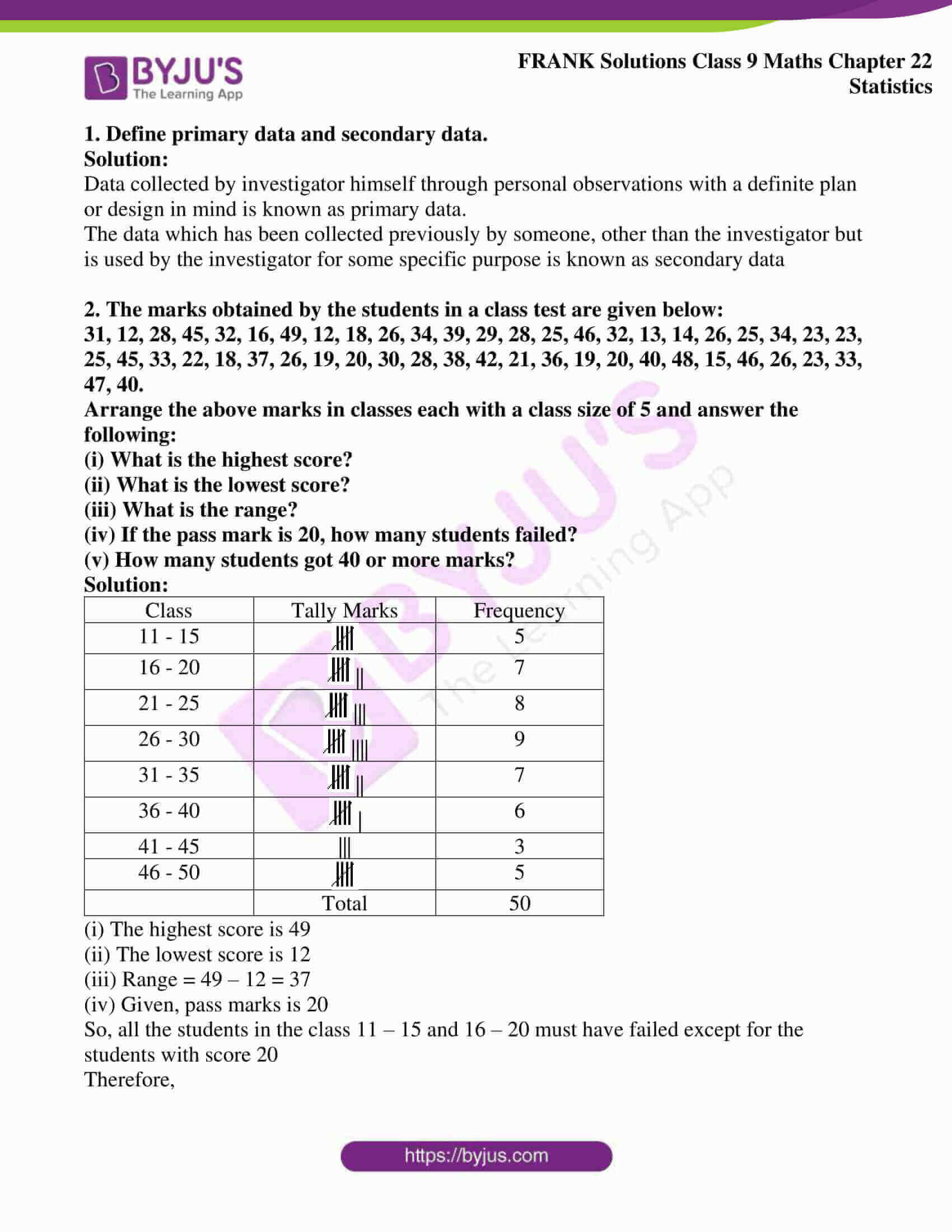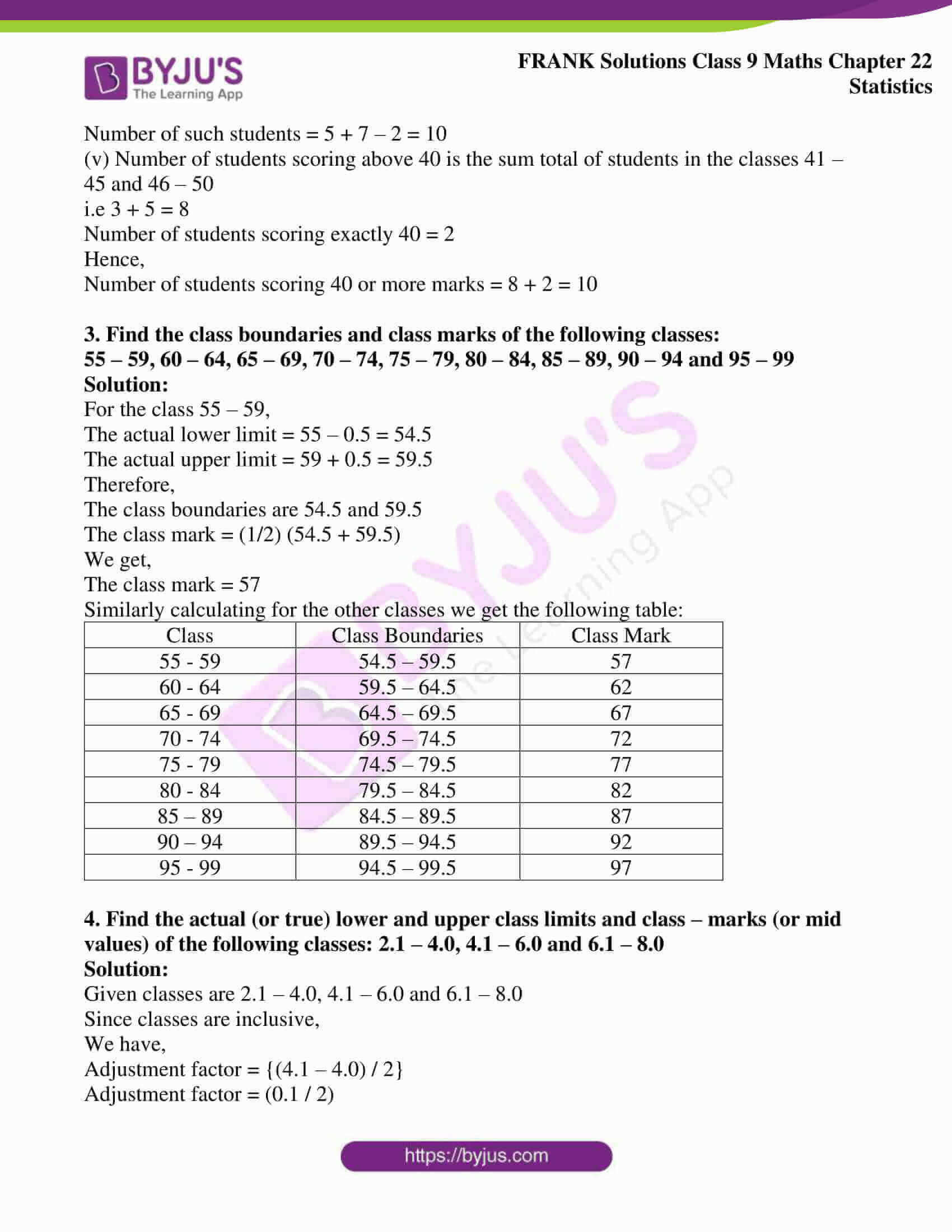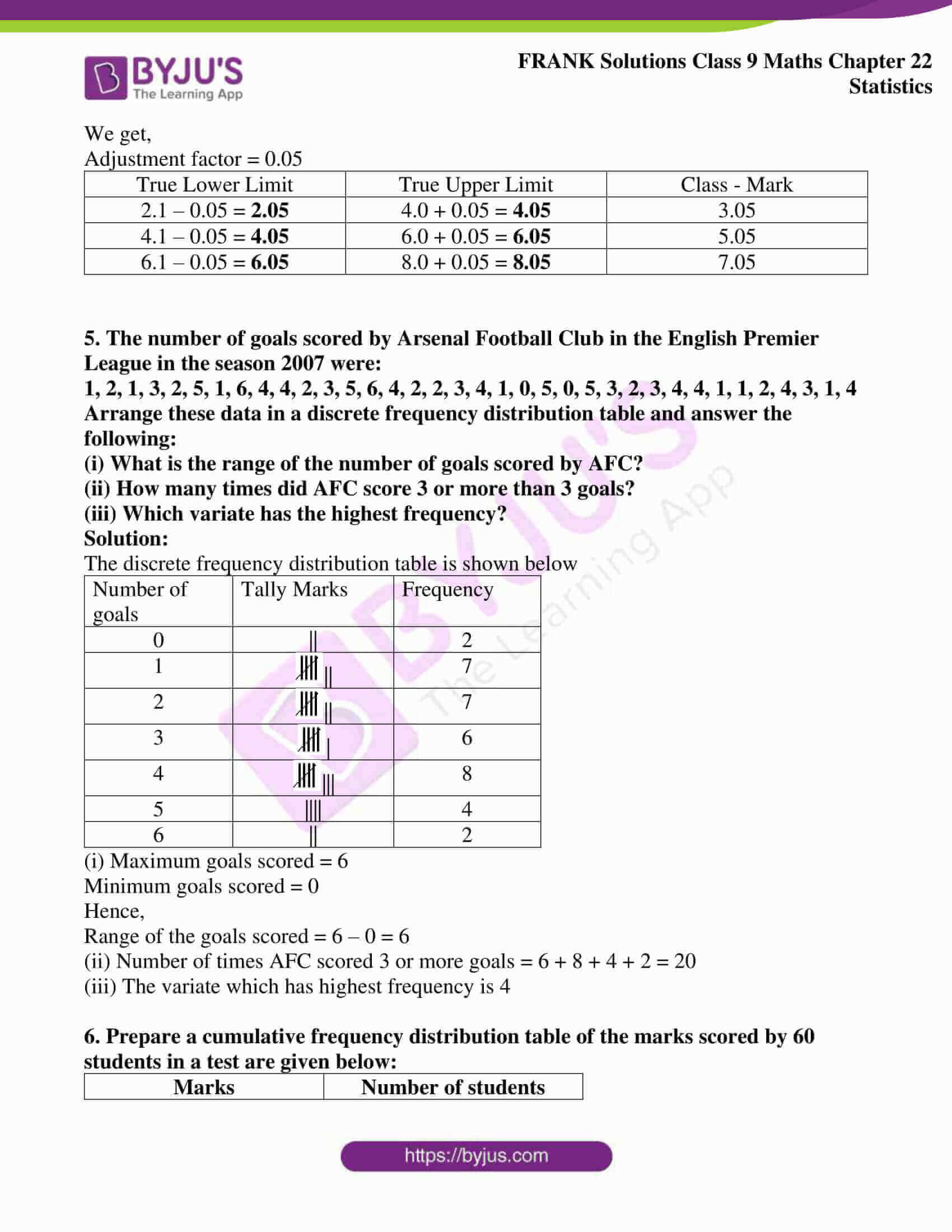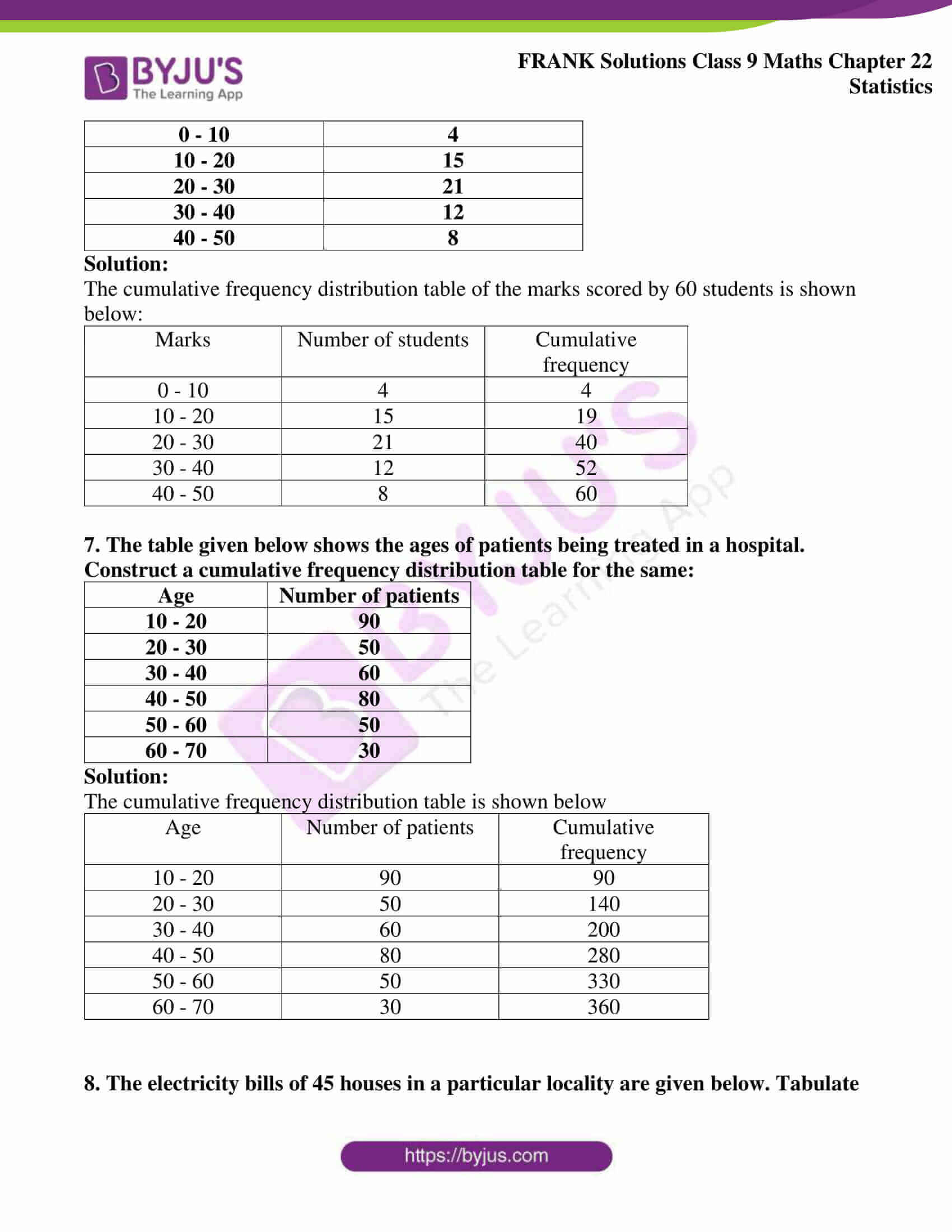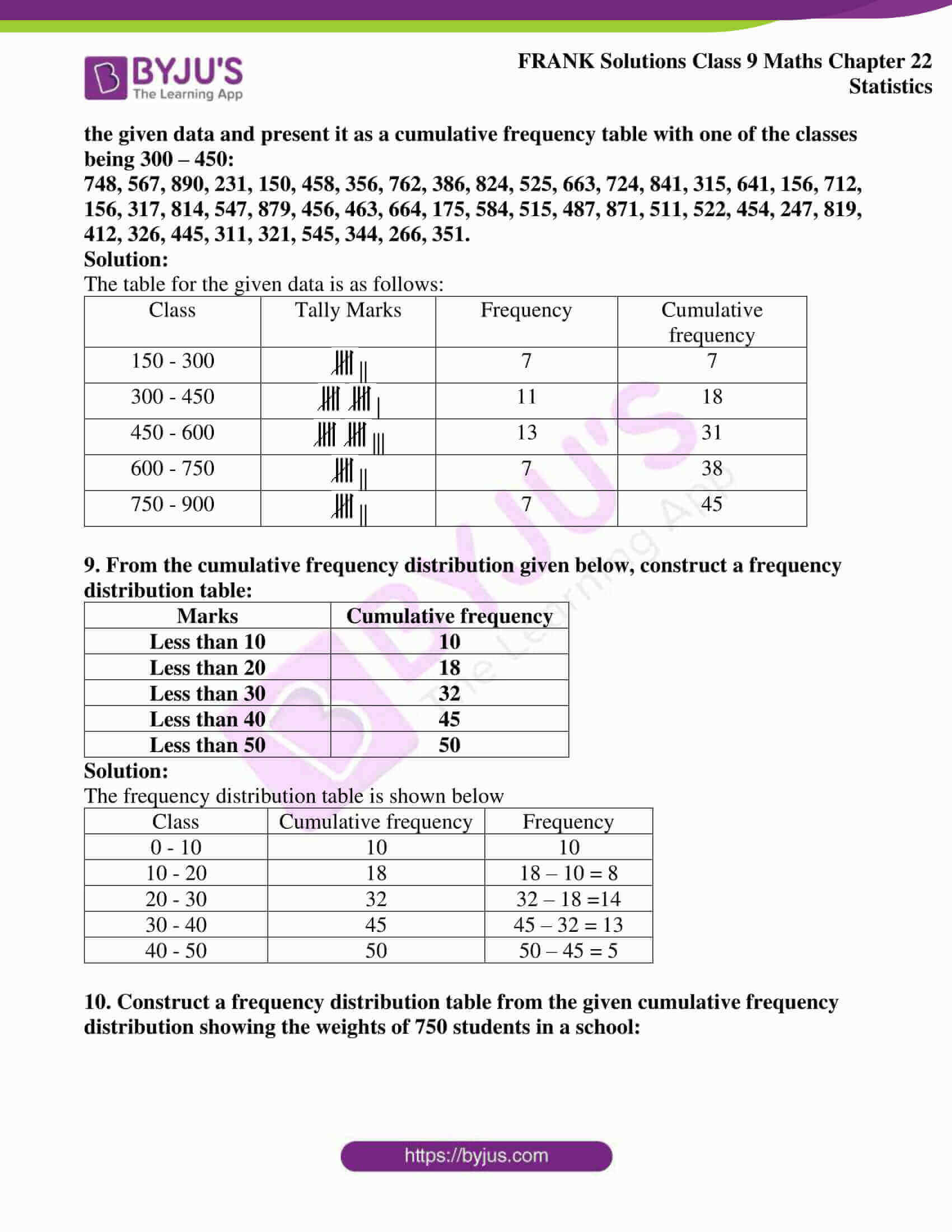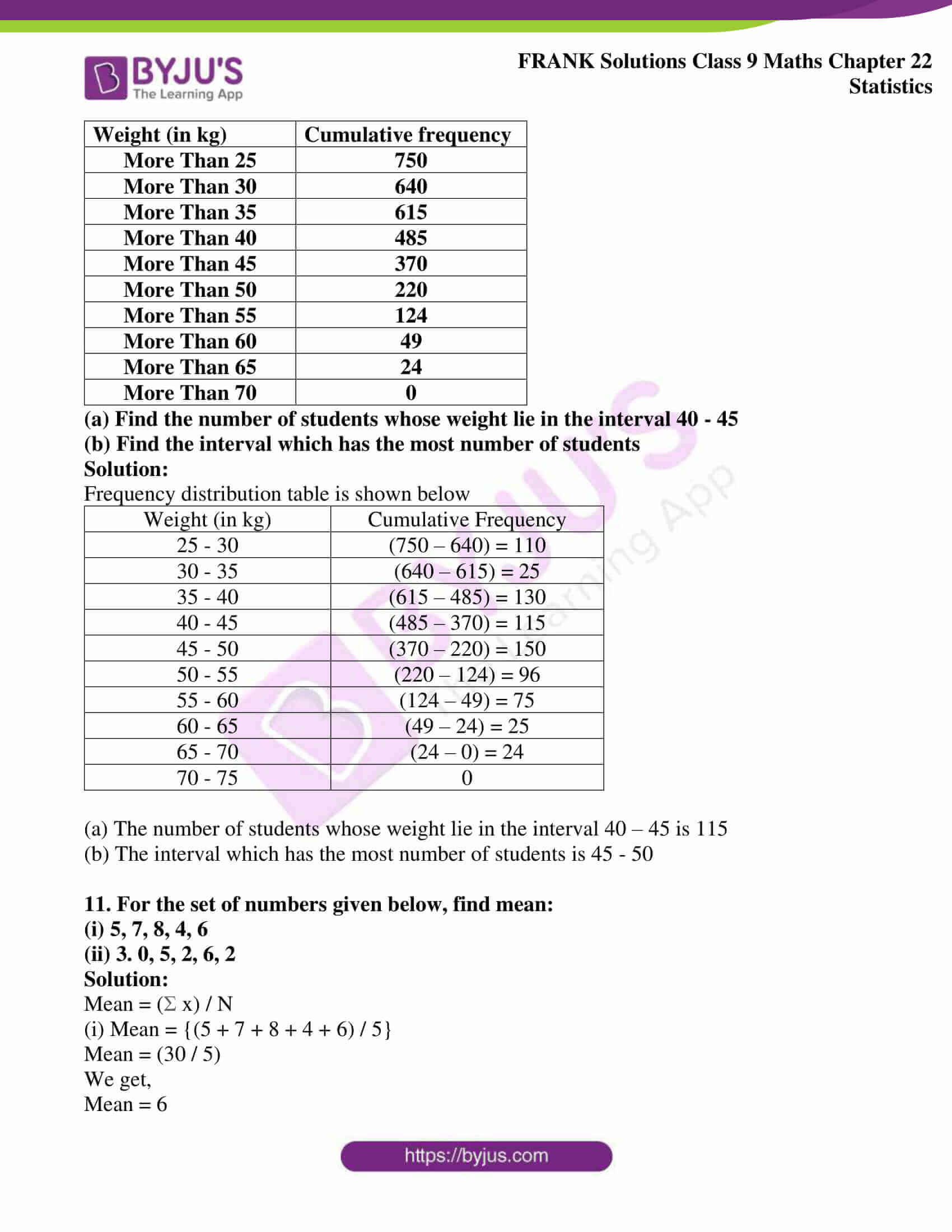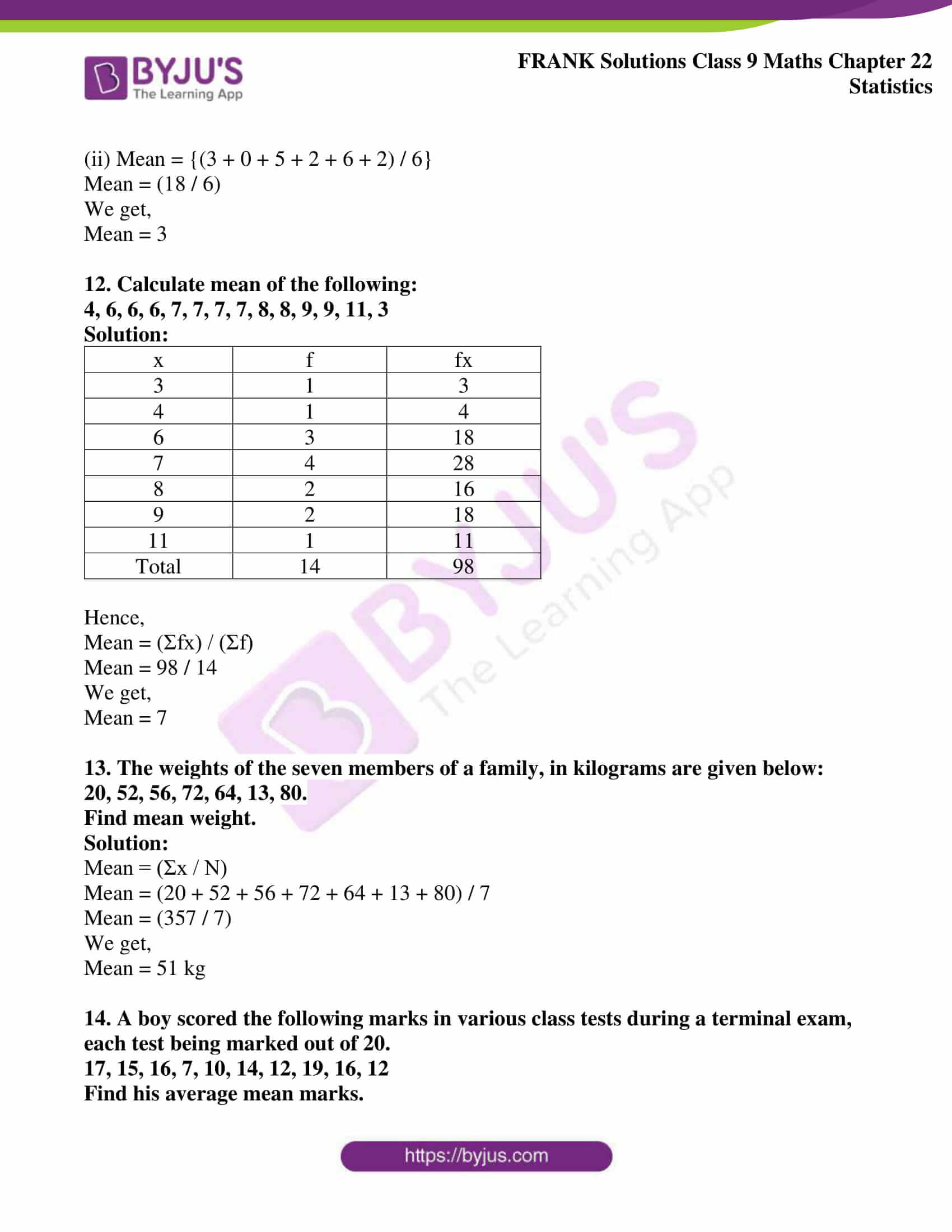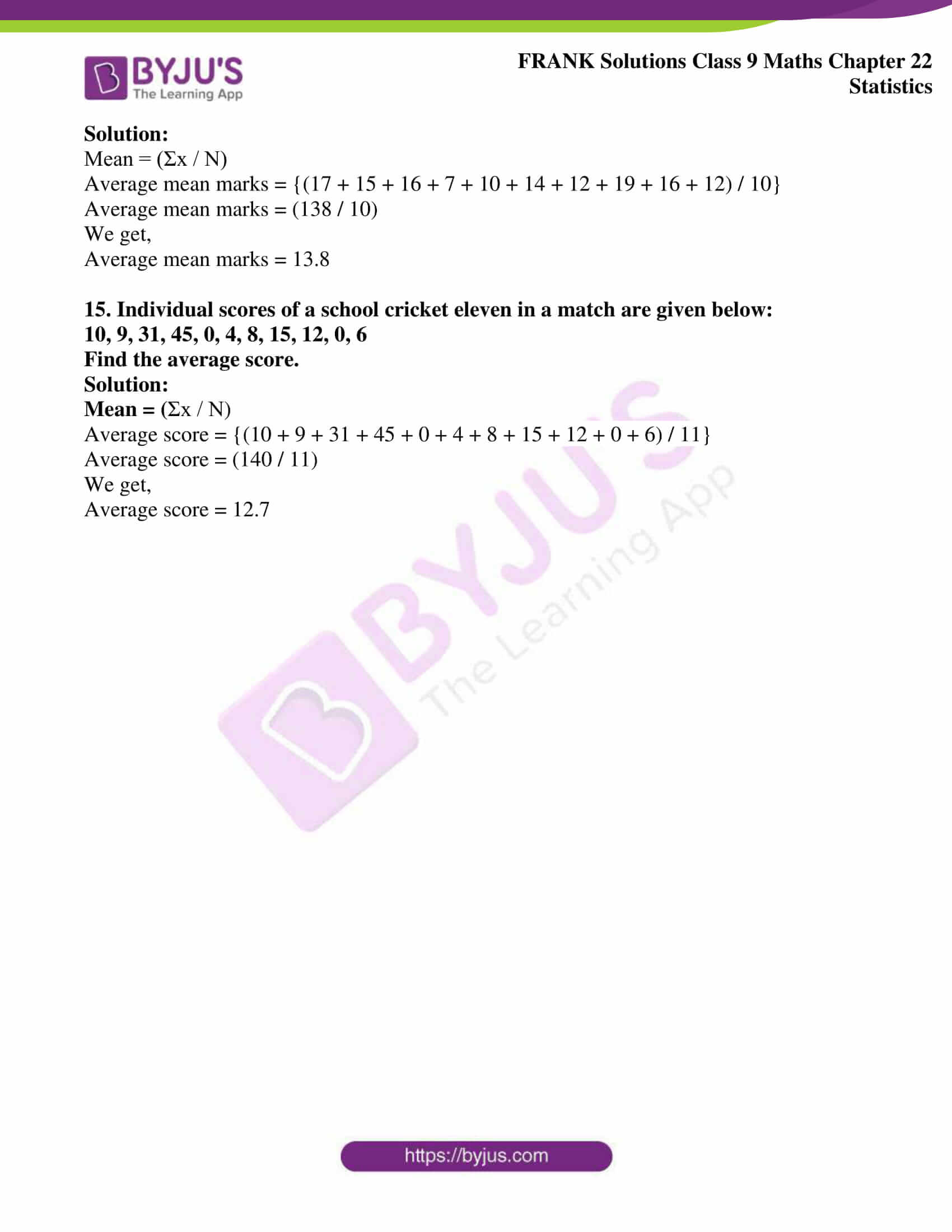## Access Frank Solutions for Class 9 Maths Chapter 22 Statistics

1. Define primary data and secondary data.

Solution:

Data collected by investigator himself through personal observations with a definite plan or design in mind is known as primary data.

The data which has been collected previously by someone, other than the investigator but is used by the investigator for some specific purpose is known as secondary data

2. The marks obtained by the students in a class test are given below:

31, 12, 28, 45, 32, 16, 49, 12, 18, 26, 34, 39, 29, 28, 25, 46, 32, 13, 14, 26, 25, 34, 23, 23, 25, 45, 33, 22, 18, 37, 26, 19, 20, 30, 28, 38, 42, 21, 36, 19, 20, 40, 48, 15, 46, 26, 23, 33, 47, 40.

Arrange the above marks in classes each with a class size of 5 and answer the following:

(i) What is the highest score?

(ii) What is the lowest score?

(iii) What is the range?

(iv) If the pass mark is 20, how many students failed?

(v) How many students got 40 or more marks?

Solution:

 Class Tally Marks Frequency 11 – 15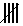5 16 – 20|| 7 21 – 25||| 8 26 – 30|||| 9 31 – 35|| 7 36 – 40| 6 41 – 45 ||| 3 46 – 505 Total 50

(i) The highest score is 49

(ii) The lowest score is 12

(iii) Range = 49 – 12 = 37

(iv) Given, pass marks is 20

So, all the students in the class 11 – 15 and 16 – 20 must have failed except for the students with score 20

Therefore,

Number of such students = 5 + 7 – 2 = 10

(v) Number of students scoring above 40 is the sum total of students in the classes 41 – 45 and 46 – 50

i.e 3 + 5 = 8

Number of students scoring exactly 40 = 2

Hence,

Number of students scoring 40 or more marks = 8 + 2 = 10

3. Find the class boundaries and class marks of the following classes:

55 – 59, 60 – 64, 65 – 69, 70 – 74, 75 – 79, 80 – 84, 85 – 89, 90 – 94 and 95 – 99

Solution:

For the class 55 – 59,

The actual lower limit = 55 – 0.5 = 54.5

The actual upper limit = 59 + 0.5 = 59.5

Therefore,

The class boundaries are 54.5 and 59.5

The class mark = (1/2) (54.5 + 59.5)

We get,

The class mark = 57

Similarly calculating for the other classes we get the following table:

 Class Class Boundaries Class Mark 55 – 59 54.5 – 59.5 57 60 – 64 59.5 – 64.5 62 65 – 69 64.5 – 69.5 67 70 – 74 69.5 – 74.5 72 75 – 79 74.5 – 79.5 77 80 – 84 79.5 – 84.5 82 85 – 89 84.5 – 89.5 87 90 – 94 89.5 – 94.5 92 95 – 99 94.5 – 99.5 97

4. Find the actual (or true) lower and upper class limits and class – marks (or mid values) of the following classes: 2.1 – 4.0, 4.1 – 6.0 and 6.1 – 8.0

Solution:

Given classes are 2.1 – 4.0, 4.1 – 6.0 and 6.1 – 8.0

Since classes are inclusive,

We have,

Adjustment factor = {(4.1 – 4.0) / 2}

Adjustment factor = (0.1 / 2)

We get,

 True Lower Limit True Upper Limit Class – Mark 2.1 – 0.05 = 2.05 4.0 + 0.05 = 4.05 3.05 4.1 – 0.05 = 4.05 6.0 + 0.05 = 6.05 5.05 6.1 – 0.05 = 6.05 8.0 + 0.05 = 8.05 7.05

5. The number of goals scored by Arsenal Football Club in the English Premier League in the season 2007 were:

1, 2, 1, 3, 2, 5, 1, 6, 4, 4, 2, 3, 5, 6, 4, 2, 2, 3, 4, 1, 0, 5, 0, 5, 3, 2, 3, 4, 4, 1, 1, 2, 4, 3, 1, 4

Arrange these data in a discrete frequency distribution table and answer the following:

(i) What is the range of the number of goals scored by AFC?

(ii) How many times did AFC score 3 or more than 3 goals?

(iii) Which variate has the highest frequency?

Solution:

The discrete frequency distribution table is shown below

 Number of goals Tally Marks Frequency 0 || 2 1|| 7 2|| 7 3| 6 4||| 8 5 |||| 4 6 || 2

(i) Maximum goals scored = 6

Minimum goals scored = 0

Hence,

Range of the goals scored = 6 – 0 = 6

(ii) Number of times AFC scored 3 or more goals = 6 + 8 + 4 + 2 = 20

(iii) The variate which has highest frequency is 4

6. Prepare a cumulative frequency distribution table of the marks scored by 60 students in a test are given below:

 Marks Number of students 0 – 10 4 10 – 20 15 20 – 30 21 30 – 40 12 40 – 50 8

Solution:

The cumulative frequency distribution table of the marks scored by 60 students is shown below:

 Marks Number of students Cumulative frequency 0 – 10 4 4 10 – 20 15 19 20 – 30 21 40 30 – 40 12 52 40 – 50 8 60

7. The table given below shows the ages of patients being treated in a hospital. Construct a cumulative frequency distribution table for the same:

 Age Number of patients 10 – 20 90 20 – 30 50 30 – 40 60 40 – 50 80 50 – 60 50 60 – 70 30

Solution:

The cumulative frequency distribution table is shown below

 Age Number of patients Cumulative frequency 10 – 20 90 90 20 – 30 50 140 30 – 40 60 200 40 – 50 80 280 50 – 60 50 330 60 – 70 30 360

8. The electricity bills of 45 houses in a particular locality are given below. Tabulate the given data and present it as a cumulative frequency table with one of the classes being 300 – 450:

748, 567, 890, 231, 150, 458, 356, 762, 386, 824, 525, 663, 724, 841, 315, 641, 156, 712, 156, 317, 814, 547, 879, 456, 463, 664, 175, 584, 515, 487, 871, 511, 522, 454, 247, 819, 412, 326, 445, 311, 321, 545, 344, 266, 351.

Solution:

The table for the given data is as follows:

 Class Tally Marks Frequency Cumulative frequency 150 – 300|| 7 7 300 – 450| 11 18 450 – 600||| 13 31 600 – 750|| 7 38 750 – 900|| 7 45

9. From the cumulative frequency distribution given below, construct a frequency distribution table:

 Marks Cumulative frequency Less than 10 10 Less than 20 18 Less than 30 32 Less than 40 45 Less than 50 50

Solution:

The frequency distribution table is shown below

 Class Cumulative frequency Frequency 0 – 10 10 10 10 – 20 18 18 – 10 = 8 20 – 30 32 32 – 18 =14 30 – 40 45 45 – 32 = 13 40 – 50 50 50 – 45 = 5

10. Construct a frequency distribution table from the given cumulative frequency distribution showing the weights of 750 students in a school:

 Weight (in kg) Cumulative frequency More Than 25 750 More Than 30 640 More Than 35 615 More Than 40 485 More Than 45 370 More Than 50 220 More Than 55 124 More Than 60 49 More Than 65 24 More Than 70 0

(a) Find the number of students whose weight lie in the interval 40 – 45

(b) Find the interval which has the most number of students

Solution:

Frequency distribution table is shown below

 Weight (in kg) Cumulative Frequency 25 – 30 (750 – 640) = 110 30 – 35 (640 – 615) = 25 35 – 40 (615 – 485) = 130 40 – 45 (485 – 370) = 115 45 – 50 (370 – 220) = 150 50 – 55 (220 – 124) = 96 55 – 60 (124 – 49) = 75 60 – 65 (49 – 24) = 25 65 – 70 (24 – 0) = 24 70 – 75 0

(a) The number of students whose weight lie in the interval 40 – 45 is 115

(b) The interval which has the most number of students is 45 – 50

11. For the set of numbers given below, find mean:

(i) 5, 7, 8, 4, 6

(ii) 3. 0, 5, 2, 6, 2

Solution:

Mean = (Σ x) / N

(i) Mean = {(5 + 7 + 8 + 4 + 6) / 5}

Mean = (30 / 5)

We get,

Mean = 6

(ii) Mean = {(3 + 0 + 5 + 2 + 6 + 2) / 6}

Mean = (18 / 6)

We get,

Mean = 3

12. Calculate mean of the following:

4, 6, 6, 6, 7, 7, 7, 7, 8, 8, 9, 9, 11, 3

Solution:

 x f fx 3 1 3 4 1 4 6 3 18 7 4 28 8 2 16 9 2 18 11 1 11 Total 14 98

Hence,

Mean = (Σfx) / (Σf)

Mean = 98 / 14

We get,

Mean = 7

13. The weights of the seven members of a family, in kilograms are given below:

20, 52, 56, 72, 64, 13, 80.

Find mean weight.

Solution:

Mean = (Σx / N)

Mean = (20 + 52 + 56 + 72 + 64 + 13 + 80) / 7

Mean = (357 / 7)

We get,

Mean = 51 kg

14. A boy scored the following marks in various class tests during a terminal exam, each test being marked out of 20.

17, 15, 16, 7, 10, 14, 12, 19, 16, 12

Find his average mean marks.

Solution:

Mean = (Σx / N)

Average mean marks = {(17 + 15 + 16 + 7 + 10 + 14 + 12 + 19 + 16 + 12) / 10}

Average mean marks = (138 / 10)

We get,

Average mean marks = 13.8

15. Individual scores of a school cricket eleven in a match are given below:

10, 9, 31, 45, 0, 4, 8, 15, 12, 0, 6

Find the average score.

Solution:

Mean = (Σx / N)

Average score = {(10 + 9 + 31 + 45 + 0 + 4 + 8 + 15 + 12 + 0 + 6) / 11}

Average score = (140 / 11)

We get,

Average score = 12.7